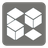Boundary Blocks
Moderate
English RU

This mission is like a subset of Visibilities.

You are given a list of strings as grid. Empty cells will be represented by '.', and the blocks - by 'X'. Blocks are not adjacent to each other vertically and horizontally.

One area consists of empty cells that are adjacent to each other vertically and horizontally. You must return the coordinates of the blocks that make the boundary.

Examples:

```set(boundary_blocks(['..X',
'.X.',
'X..'])) == {(0, 2), (1, 1), (2, 0)}
set(boundary_blocks(['...',
'.X.',
'X..'])) == set()
set(boundary_blocks(['X.X.',
'.X..',
'..X.',
'....'])) == {(0, 0), (0, 2), (1, 1)}
```Input: A list of strings.

Output: An iterable of tuples of two integers (In row, column order).

Preconditions:

• 3 ≤ len(grid) ≤ 20
• 3 ≤ len(grid) ≤ 20
• all(set(row) <= {'.', 'X'} for row in grid)